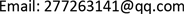﻿ Banach空间上凸集的k凸性 K-Convexity of Convex Sets in Banach Spaces

Pure Mathematics
Vol. 08  No. 05 ( 2018 ), Article ID: 27042 , 4 pages
10.12677/PM.2018.85077

K-Convexity of Convex Sets in Banach Spaces

Jing Zhang

Northeast Forestry University, Harbin HeilongjiangReceived: Sep. 7th, 2018; accepted: Sep. 23rd, 2018; published: Sep. 30th, 2018ABSTRACT

This paper breaks through the shackles of the unit ball, and generalizes the convexity theory of Banach space to the convex set which is not empty internally. It is more extensive than the k-convexity research of Banach Spaces.

Keywords:Convexity Theory, K-Convexity, Banach Spaces

Banach空间上凸集的k凸性1. 引言

1977年，Sullian引入了k一致凸空间的概念，开始了对Banach空间的k凸性的研究  。Banach空间的各种k凸性有一个共同的特点，即以Banach空间的单位球作为研究对象  。本文把Banach空间的凸性理论推广到内部不空的凸集上。首先，引入下面定义 

2. 主要结果

1) 当 ${\mu }_{A}\left({\sum }_{i=1}^{k+1}{x}_{i}\right)={\sum }_{i=1}^{k+1}{\mu }_{A}\left({x}_{i}\right),\text{\hspace{0.17em}}{x}_{1},{x}_{2},\cdots ,{x}_{k+1}\in \partial A$ 时，存在不全为0的 $k+1$ 个实数 ${r}_{1},{r}_{2},\cdots ,{r}_{k+1}$ ，使得 ${\sum }_{i=1}^{k+1}{r}_{i}{x}_{i}=0$ 成立。其中 ${\mu }_{A}$ 是A上的Minkowski泛函。

2) 任意 $x\in \partial \left(A\right)$ ，则x是A的k端点。

3) A是k严格凸集。

${x}_{1},{x}_{2},\cdots ,{x}_{k+1}\in A$$0<{t}_{i}<1$${\sum }_{i=1}^{k+1}{t}_{i}=1$ ，使得 ${z}_{0}={\sum }_{i=1}^{k+1}{t}_{i}{x}_{i}$

${\mu }_{A}$ 为A上的Minkowski泛函，且 $0\in \mathrm{int}\left(A\right)$ ，故 ${\mu }_{A}$ 为X上的连续泛函，且 $\mathrm{int}\left(A\right)=\left\{x:{\mu }_{A}\left(x\right)<1\right\}$ ，且有 $\stackrel{¯}{\mathrm{int}\left(A\right)}=\stackrel{¯}{A}$ 成立。所以

$1={\mu }_{A}\left({z}_{0}\right)={\mu }_{A}\left(\underset{i=1}{\overset{k+1}{\sum }}{t}_{i}{x}_{i}\right)\le \underset{i=1}{\overset{k+1}{\sum }}{t}_{i}{\mu }_{A}\left({x}_{i}\right)\le \underset{i=1}{\overset{k+1}{\sum }}{t}_{i}=1$

$\begin{array}{c}{\mu }_{A}\left({z}_{0}\right)={\mu }_{A}\left({\left({t}_{1}+\cdots +{t}_{k+1}\right)}^{k}{z}_{0}\right)={\mu }_{A}\left({\left({t}_{1}+\cdots +{t}_{k+1}\right)}^{k}\left(\underset{i=1}{\overset{k+1}{\sum }}{t}_{i}{x}_{i}\right)\right)\\ ={\mu }_{A}\left({t}_{1}^{k+1}{x}_{1}+\cdots +{t}_{k+1}^{k+1}{x}_{k+1}+\cdots +k!{t}_{1}{t}_{2}\cdots {t}_{k+1}\left({x}_{1}+\cdots +{x}_{k+1}\right)\right)\\ <{t}_{1}^{k+1}+\cdots +{t}_{k+1}^{k+1}+\cdots +k!{t}_{1}{t}_{2}\cdots {t}_{k+1}\left(k+1\right)\\ ={\left({t}_{1}+\cdots +{t}_{k+1}\right)}^{k}\left({t}_{1}+\cdots +{t}_{k+1}\right)=1\end{array}$

${\mu }_{A}\left({z}_{0}\right)=1$ ，即 $1<1$ ，矛盾。故 ${\mu }_{A}\left({\sum }_{i=1}^{k+1}{x}_{i}\right)={\sum }_{i=1}^{k+1}{\mu }_{A}\left({x}_{i}\right)$ 成立。但不存在一组不全为0的实数 ${r}_{1},{r}_{2},\cdots ,{r}_{k+1}$ ，使得 ${\sum }_{i=1}^{k+1}{r}_{i}{x}_{i}=0$ ，同1)矛盾，故2)成立。

2)⟹3)任意 $x\in \partial \left(A\right)$ ，及实泛函f，满足 $f\left(x\right)\ge \mathrm{sup}f\left(\mathrm{int}A\right)$ ，任取 ${x}_{1},{x}_{2},\cdots ,{x}_{k+1}\in \left\{y:f\left(y\right)=f\left(x\right)\right\}$ ，令 $z=\frac{1}{k+1}\left({\sum }_{i=1}^{k+1}{x}_{i}\right)$ ，易证 $z\in \left\{y:f\left(y\right)=f\left(x\right)\right\}$ ，则 $z\in \partial A$ (事实上，假设 $z\in \mathrm{int}A$ ，则存在 $r\in R$ ，且 $r>1$

s.t $rz\in \mathrm{int}\left(A\right)$ ，由 $f\left(x\right)\ge \mathrm{sup}f\left(\mathrm{int}A\right)$$\stackrel{¯}{\mathrm{int}A}=\stackrel{¯}{A}$$f\left(x\right)\ge \mathrm{sup}f\left(A\right)$$r\left(z\right)\in A$ ，故 $f\left(x\right)=f\left(z\right)\ge f\left(rz\right)$ ，故 $1\ge r$ ，矛盾)通过2)知z是A的k端点。故不存在一组不全为0的实数 ${r}_{1},{r}_{2},\cdots ,{r}_{k+1}$ ，使得 ${\sum }_{i=1}^{k+1}{r}_{i}{x}_{i}=0$ ，故 $\left\{y=f\left(y\right)=f\left(x\right)\right\}$ 张成的实线性子空间维数不超过k。故3)成立。

3)⟹1)假设1)不成立，则存在 ${x}_{1},{x}_{2},\cdots ,{x}_{k+1}\in \partial A$ ，使得 ${\mu }_{A}\left({\sum }_{i=1}^{k+1}{x}_{i}\right)={\sum }_{i=1}^{k+1}{\mu }_{A}\left({x}_{i}\right)$ ，但不存在一组不全为0的实数 ${r}_{1},{r}_{2},\cdots ,{r}_{k+1}$ ，使得 ${\sum }_{i=1}^{k+1}{r}_{i}{x}_{i}=0$ ，令 ${x}_{0}=\frac{1}{k+1}{\sum }_{i=1}^{k+1}{x}_{i}⇒{\mu }_{A}\left({x}_{0}\right)=\frac{1}{k+1}{\sum }_{i=1}^{k+1}{\mu }_{A}\left({x}_{i}\right)=1$ ，故 ${x}_{0}\in \partial A$ 。由分离定理存在实泛函f，使得 $f\left({x}_{0}\right)\ge \mathrm{sup}f\left(\mathrm{int}A\right)$ ，故 $f\left({x}_{i}\right)=f\left({x}_{0}\right),i=1,2,\cdots ,k+1$ ，因此 ${\left\{{x}_{i}\right\}}_{i=1}^{k+1}\subset \left\{y:f\left(y\right)=f\left({x}_{0}\right)\right\}$ 。由3)知 $\left\{y=f\left(y\right)=f\left({x}_{0}\right)\right\}$ 张成的实线性子空间维数不超过k，矛盾。故1)成立。

$A\left({x}_{1},{x}_{2},\cdots ,{x}_{k+1}\right)=\mathrm{sup}\left\{‖\begin{array}{cccc}1& 1& \cdots & 1\\ {f}_{1}\left({x}_{1}\right)& {f}_{1}\left({x}_{2}\right)& \cdots & {f}_{1}\left({x}_{k+1}\right)\\ ⋮& ⋮& \ddots & ⋮\\ {f}_{k+1}\left({x}_{1}\right)& {f}_{k+1}\left({x}_{1}\right)& \cdots & {f}_{k+1}\left({x}_{k+1}\right)\end{array}‖:{f}_{i}\in S\left({X}^{*}\right)\right\}<\epsilon$

$\left\{\left({x}_{1}^{\left(n\right)},{x}_{2}^{\left(n\right)},\cdots ,{x}_{k+2}^{\left(n\right)}\right)|n=1,2,\cdots \right\}$ 是属于A边界的 $\left(k+2\right)$ 个元列，且存在实数列 ${\left\{{\delta }_{n}\right\}}_{n=1}^{\infty }$${\delta }_{n}\to 0\left(n\to \infty \right)$${B}_{{\delta }_{n}}\left(\frac{1}{k+1}\left({x}_{1}+{x}_{2}+\cdots +{x}_{k+1}\right)\right)\not\subset A$ 成立，则存在实数列 ${\left\{{{\delta }^{\prime }}_{n}\right\}}_{n=1}^{\infty }$${{\delta }^{\prime }}_{n}\to 0\left(n\to \infty \right)$ ，使得 ${B}_{{{\delta }^{\prime }}_{n}}\left(\frac{1}{k+1}\left({x}_{1}+{x}_{2}+\cdots +{x}_{k+1}\right)\right)\not\subset A$ 成立。(事实上，假设上述结论不成立，则存在 $\delta >0$ ，使得 ${B}_{\delta }\left(\frac{1}{k+1}\left({x}_{1}+{x}_{2}+\cdots +{x}_{k+1}\right)\right)\subset A$ 成立。即 $\frac{1}{k+1}\left({x}_{1}+{x}_{2}+\cdots +{x}_{k+1}\right)\in \mathrm{int}\left(A\right)$ ，不失一般性，不妨令 $0\in \mathrm{int}\left(A\right)$ ，令 ${\mu }_{A}$ 为A上的Minkowski泛函，则 ${\mu }_{A}$ 连续且 $\mathrm{int}\left(A\right)=\left\{x:{\mu }_{A}<1\right\}$ ，则

$\begin{array}{l}{\mu }_{A}\left(\frac{1}{k+2}\left({x}_{1}+{x}_{2}+\cdots +{x}_{k+2}\right)\right)={\mu }_{A}\left(\frac{k+1}{k+2}\left(\frac{{x}_{1}^{\left({n}_{k}\right)}+\cdots +{x}_{k+1}^{\left({n}_{k}\right)}}{k+1}\right)+\frac{1}{k+2}{x}_{k+2}^{\left({n}_{k}\right)}\right)\\ =\frac{k+1}{k+2}{\mu }_{A}\left(\frac{1}{k+1}\left({x}_{1}^{\left({n}_{k}\right)}+\cdots +{x}_{k+2}^{\left({n}_{k}\right)}\right)+\frac{1}{k+2}{x}_{k+2}^{\left({n}_{k}\right)}\right)=m<1\end{array}$

${y}_{{n}_{k}}=\frac{1}{k+2}\left({x}_{1}^{\left({n}_{k}\right)}+\cdots +{x}_{k+2}^{\left({n}_{k}\right)}\right)$ ，由 ${\mu }_{A}$ 的连续性知，存在0点的领域 ${B}_{{\delta }_{1}}\left(0\right)$ ，使得 ${\mu }_{A}\left({B}_{{\delta }_{1}}\left(0\right)\right)<\frac{1}{2}\left(1-m\right)$ ，取 ${y}_{{n}_{k}}$ 的领域 ${y}_{{n}_{k}}+{B}_{{\delta }_{1}}\left(0\right)$ ，当 $y\in {y}_{{n}_{k}}+{B}_{{\delta }_{1}}\left(0\right)$ 时， ${\mu }_{A}\left(y\right)\le {\mu }_{A}\left(y-{y}_{{n}_{k}}\right)+{\mu }_{A}\left({y}_{{n}_{k}}\right)\le \frac{1}{2}\left(1-m\right)+m<1$ ，故 ${y}_{{n}_{k}}+{B}_{{\delta }_{1}}\left(0\right)\subset \mathrm{int}\left(A\right)\subset A$ 。这与存在实数列 ${\left\{{\delta }_{n}\right\}}_{n=1}^{\infty }$${\delta }_{n}\to 0\left(n\to \infty \right)$${B}_{{\delta }_{n}}\left(\frac{1}{k+1}\left({x}_{1}+{x}_{2}+\cdots +{x}_{k+1}\right)\right)\not\subset A$ 矛盾。)由A的k一致凸性知 $A\left({x}_{1}^{\left(n\right)},\cdots ,{x}_{k+1}^{\left(n\right)}\right)\to 0\left(n\to \infty \right)$ ，同理当 $\left(1\le i\le k+2\right)$ 时， $A\left({x}_{1}^{\left(n\right)},\cdots ,{x}_{i-1}^{\left(n\right)},{x}_{i+1}^{\left(n\right)},\cdots ,{x}_{k+1}^{\left(n\right)}\right)\to 0\left(n\to \infty \right)$

$\begin{array}{l}\mathrm{sup}\left\{‖\begin{array}{cccc}1& 1& \cdots & 1\\ {f}_{1}\left({x}_{1}\right)& {f}_{1}\left({x}_{2}\right)& \cdots & {f}_{1}\left({x}_{k+1}\right)\\ ⋮& ⋮& \ddots & ⋮\\ {f}_{k}\left({x}_{k}\right)& {f}_{k}\left({x}_{k}\right)& \cdots & {f}_{k}\left({x}_{k+1}\right)\end{array}‖:{f}_{i}\in S{\left(X,{‖\text{ }\cdot \text{ }‖}_{1}\right)}^{*}\right\}\\ =‖{f}_{1}‖\cdots ‖{f}_{k}‖\mathrm{sup}\left\{‖\begin{array}{cccc}1& 1& \cdots & 1\\ \frac{{f}_{1}}{{f}_{1}}\left({x}_{1}\right)& \frac{{f}_{1}}{{f}_{1}}\left({x}_{2}\right)& \cdots & \frac{{f}_{1}}{{f}_{1}}\left({x}_{k+1}\right)\\ ⋮& ⋮& \ddots & ⋮\\ \frac{{f}_{k}}{{f}_{k}}\left({x}_{k}\right)& \frac{{f}_{k}}{{f}_{k}}\left({x}_{k}\right)& \cdots & \frac{{f}_{k}}{{f}_{k}}\left({x}_{k+1}\right)\end{array}‖:{f}_{i}\in S{\left(X,{‖\text{ }\cdot \text{ }‖}_{1}\right)}^{*}\right\}\\ \le ‖{f}_{1}‖\cdots ‖{f}_{k}‖A\left({x}_{1},\cdots ,{x}_{k+1}\right)\le {\left(\frac{1}{{m}^{\prime }}\right)}^{k}{‖{f}_{1}‖}_{1}\cdots {‖{f}_{k}‖}_{1}\frac{\epsilon }{M}=\frac{1}{M}{\left(\frac{1}{{m}^{\prime }}\right)}^{k}\epsilon \end{array}$

$\left(X,{‖\text{ }\cdot \text{ }‖}_{1}\right)$ 是k一致凸。

K-Convexity of Convex Sets in Banach Spaces[J]. 理论数学, 2018, 08(05): 580-583. https://doi.org/10.12677/PM.2018.85077

1. 1. 方习年. Banach空间的凸性和光滑性及其应用[J]. 安徽工程大学学报, 2001, 16(3): 1-8.

2. 2. 乌日娜. Banach空间的某些凸性、光滑性与可凹性的研究[D]: [硕士学位论文]. 内蒙古: 内蒙古师范大学, 2008.

3. 3. 商绍强. Banach空间上凸集的凸性[J]. 应用泛函分析学报, 2009, 11(2): 170-177.